# The MI Procedure

### Imputer’s Model Versus Analyst’s Model

Multiple imputation inference assumes that the model you used to analyze the multiply imputed data (the analyst’s model) is the same as the model used to impute missing values in multiple imputation (the imputer’s model). But in practice, the two models might not be the same (Schafer 1997, p. 139).

Schafer (1997, pp. 139–143) provides comprehensive coverage of this topic, and the following example is based on his work.

Consider a trivariate data set with variables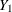and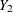fully observed, and a variable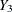with missing values. An imputer creates multiple imputations with the model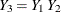. However, the analyst can later use the simpler model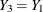. In this case, the analyst assumes more than the imputer. That is, the analyst assumes there is no relationship between variablesand.

The effect of the discrepancy between the models depends on whether the analyst’s additional assumption is true. If the assumption is true, the imputer’s model still applies. The inferences derived from multiple imputations will still be valid, although they might be somewhat conservative because they reflect the additional uncertainty of estimating the relationship betweenand.

On the other hand, suppose that the analyst models, and there is a relationship between variablesand. Then the modelwill be biased and is inappropriate. Appropriate results can be generated only from appropriate analyst models.

Another type of discrepancy occurs when the imputer assumes more than the analyst. For example, suppose that an imputer creates multiple imputations with the model, but the analyst later fits a model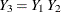. When the assumption is true, the imputer’s model is a correct model and the inferences still hold.

On the other hand, suppose there is a relationship betweenand. Imputations created under the incorrect assumption that there is no relationship betweenandwill make the analyst’s estimate of the relationship biased toward zero. Multiple imputations created under an incorrect model can lead to incorrect conclusions.

Thus, generally you should include as many variables as you can when doing multiple imputation. The precision you lose with included unimportant predictors is usually a relatively small price to pay for the general validity of analyses of the resultant multiply imputed data set (Rubin 1996). But at the same time, you need to keep the model building and fitting feasible (Barnard and Meng 1999, pp. 19–20).

To produce high-quality imputations for a particular variable, the imputation model should also include variables that are potentially related to the imputed variable and variables that are potentially related to the missingness of the imputed variable (Schafer 1997, p. 143).

Similar suggestions were also given by Van Buuren, Boshuizen, and Knook (1999, p. 687). They recommend that the imputation model include three sets of covariates: variables in the analyst’s model, variables associated with the missingness of the imputed variable, and variables correlated with the imputed variable. They also recommend the removal of the covariates not in the analyst’s model if they have too many missing values for observations with missing imputed variables.

Note that it is good practice to include a description of the imputer’s model with the multiply imputed data set (Rubin 1996, p. 479). That way, the analysts will have information about the variables involved in the imputation and which relationships among the variables have been implicitly set to zero.